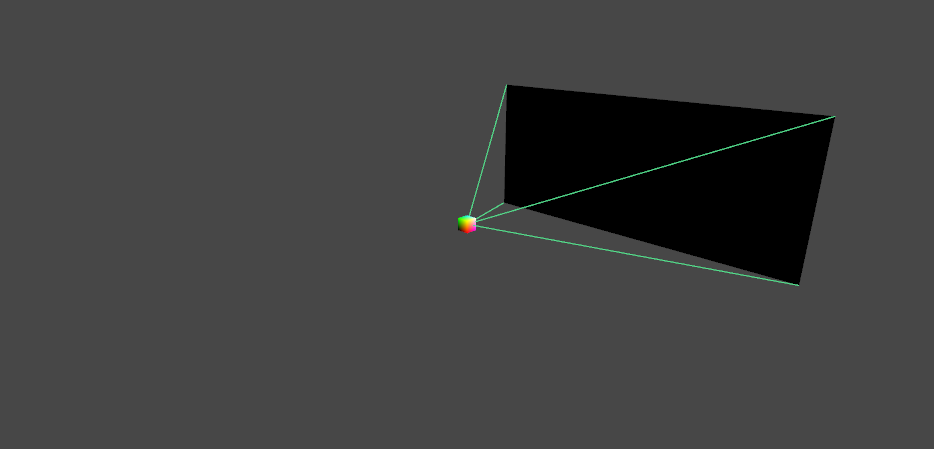# SOLVEDMatrix4

Hi folks,

I want to build a perspective matrix using native Cinema objects. I read in the manual here that the first row in a Matrix4 is a "constant row vector at the top... which cannot be changed, due to the fact that Cinema’s transforms do not support projections".

Can someone educate me a little further on this. I just want to know if I can make a perspective matrix using Cinema's native Matrix4 object. But it sounds like we can't touch the first row at all? Is this really not possible?

WP.

Hello @wickedp,

Thank you for reaching out to us. Well, the sentence

Cinema’s transforms do not support projections ...

is a tad absolute, there are matrix types in Cinema 4D which support non-linear transforms, but this manual has been written for Python and the classic API type `Matrix` for which this statement holds true: You cannot carry out non-linear transforms with this type, as for example projection transforms, due to the fact that you can only write 12 of the 16 values.

With the maxon API types this does not hold true, with `maxon::SqrMat4` for example, you can define 4x4 transforms of any type to your heart's content. On top of that comes the fact that the old classic API types `Matrix` and `Vector` are only aliases for the maxon API types now, i.e., that everyone is already using the new system under the hood. But I did not mention this then in the Python manual, as this is effectively irrelevant in Python, as the maxon API is there not mature enough yet, to truly use these interfaces.

Below you will find some code showing how to use the templated type `SqrMat4`. But I would also point out that you usually do not have to do projections yourself in this manner, as at least for Cinema 4D cameras, all the necessary projection work can be done with BaseView.

Cheers,
Ferdinand

The code:

``````// The whole vector/matrix type system is templated in the maxon API. And the old classic API
// types are only aliases for that system. You can define matrices of different shape, precision,
// and type with this new system, e.g., you could define a 4x4 matrix which stores boolean or real
// values. For the case of real values, you could choose between half, single, or double
// precision. When using these more modern interfaces, you can also carry out non-linear
// transforms, i.e., actually define all 16 fields of a 4x4 matrix, instead of only the 12 ones
// used to define linear transforms.

// Because of that, it is sometimes useful to define type aliases for the exact type one requires,
// as for example this type alias for a 4x4 double precision matrix. But this is not really
// required in any case, and for this case there is also an alias for at least that vector type.
using Matrix44D = maxon::SqrMat4<maxon::Vec4<maxon::Float64>>;
// Matrix44D is the same as maxon::SqrMat4 <maxon::Vector4d64>, i.e., Vector4d64 is the same as
// maxon::Vec4<maxon::Float64>.

// Define a 4x4 matrix with all 16 components, making up a linear transform, here just the
// identity matrix for clarity.
const maxon::SqrMat4 <maxon::Vector4d64> lTransform = maxon::SqrMat4 <maxon::Vector4d64>(
maxon::Vector4d64(1, 0, 0, 0),
maxon::Vector4d64(0, 1, 0, 0),
maxon::Vector4d64(0, 0, 1, 0),
maxon::Vector4d64(0, 0, 0, 1));

// Define a 4x4 matrix with all 16 components, making up a non-linear transform, e.g., a
// projection matrix (this thing does not make much sense and is only for illustration purposes).
const maxon::SqrMat4 <maxon::Vector4d64> nlTransform = maxon::SqrMat4 <maxon::Vector4d64>(
maxon::Vector4d64(1, 0, 0, 0),
maxon::Vector4d64(0, 1, 0, 0),
maxon::Vector4d64(0, 0, 1,-1),
maxon::Vector4d64(0, 0, 0, 0));

// A point in R³.
const maxon::Vector64 p = maxon::Vector64(1, 2, 3);

// Printing out the data.
ApplicationOutput("lTransform = @", lTransform);
ApplicationOutput("nlTransform = @", nlTransform);
ApplicationOutput("p = @", p);

// Using both transforms.
ApplicationOutput("linear transform * p = @", lTransform * p);
ApplicationOutput("non-linear transform * p = @", nlTransform * p);
``````

The output:

``````
lTransform = ((1.000,0.000,0.000,0.000),(0.000,1.000,0.000,0.000),(0.000,0.000,1.000,0.000),(0.000,0.000,0.000,1.000))
nlTransform = ((1.000,0.000,0.000,0.000),(0.000,1.000,0.000,0.000),(0.000,0.000,1.000,-1.000),(0.000,0.000,0.000,0.000))
p = (1.000,2.000,3.000)
linear transform * p = (1.000,2.000,3.000)
non-linear transform * p = (-0.333,-0.667,-1.000)
``````

Hello @WickedP,

without any further questions or other postings, we will consider this topic as solved and flag it as such by Friday, 17/06/2022.

Ferdinand

Hi @ferdinand,

sorry, I'm still trying to figure this out my end. What I'm trying to do isn't working, and I can't for the life of me see why.

By all means close if you need, 'tis not a problem.

WP.

Hey @wickedp,

I know that I sometimes apply pressure in the forum so that things are keept "in order" but this is not an end in itself. A thread can stay open if you struggle with understanding the problem and we will also try to provide support on subjects which are formally out of support but so central that developers encounter them all the time, e.g., algebraic stuff.

I will leave this thread open for now and simply ask in 14 days cycle if we can close it. If you think the topic is then still relevant to you, you are welcome to postpone the closing of the topic a few times by simply stating that this is not yet resolved for you.

If there are still algebraic or other conceptual questions, I would encourage you to ask them here, I am sure either us in a reduced support fashion or the community can give some pointers.

Cheers,
Ferdinand

Thanks @ferdinand,

I've been trying to remove a matrix math library from my software, but having never been taught any of this before (code that is), I was fumbling around in the dark on how to re-assemble things. But I've managed to end up with a blend of C4D and my own functionality, which is fine.

The image below probably won't mean much to you, but it tells me it's working correctly again.We can probably mark as solved.

WP.